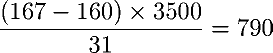Next: Making weight charts Up: Pencil and Paper Previous: Calculating weight loss

Calculating calorie deficit

To calculate the average daily calorie shortfall (or excess) for a month, take the total weight loss (or gain) that month (for example 167-160=7 pounds) measured from the trend numbers, multiply by 3500 to obtain the total calories burned from fat (or stored there), then divide by the number of days in the month (31 for July, for example) to give the average daily calorie shortfall or excess. If you started July with the trend at 167 and ended it with the trend at 160, then each day you ate an average offewer calories than you burned.

By John Walker